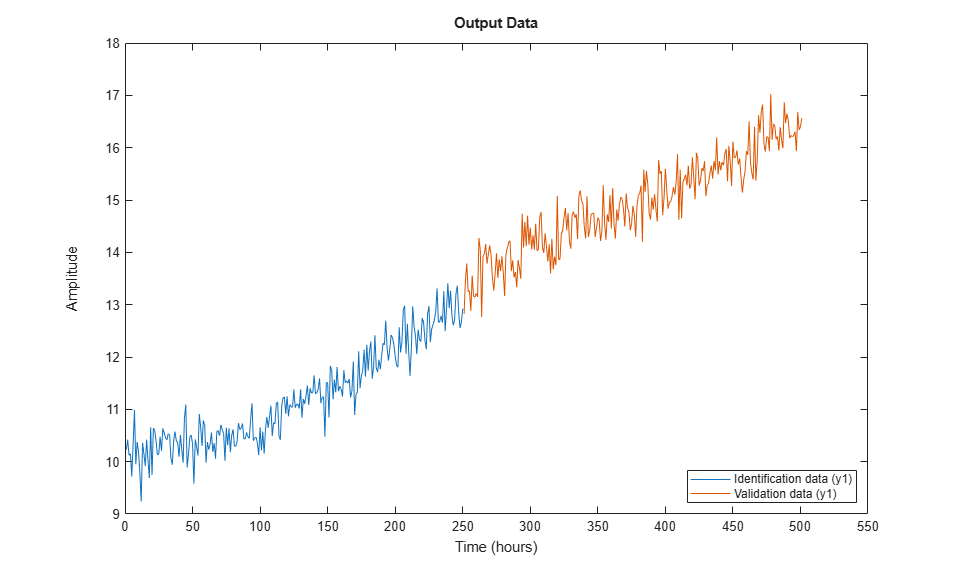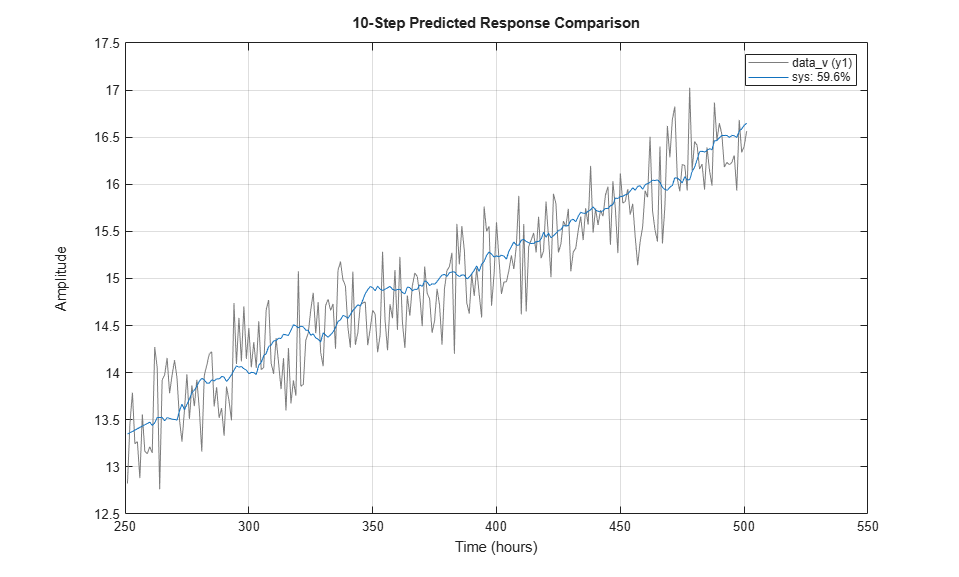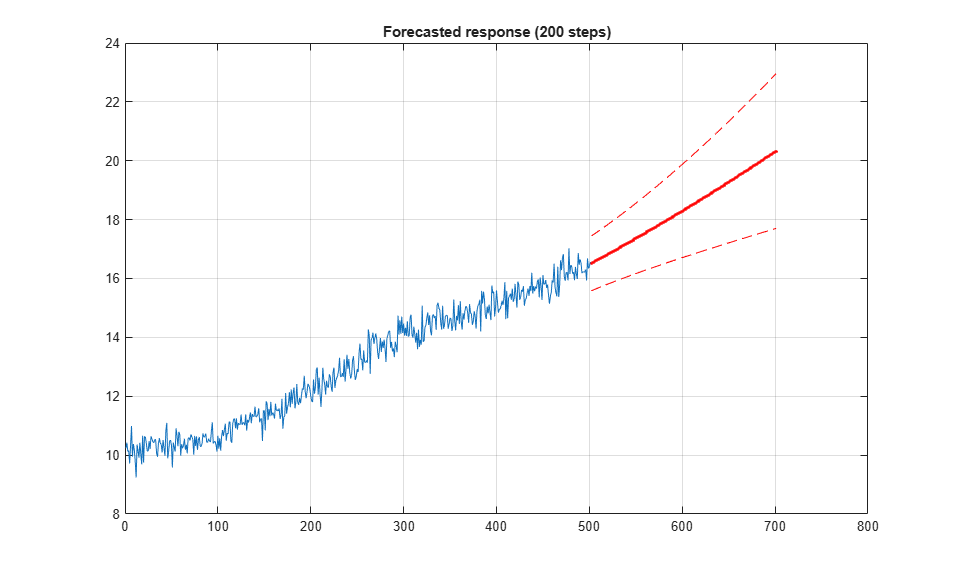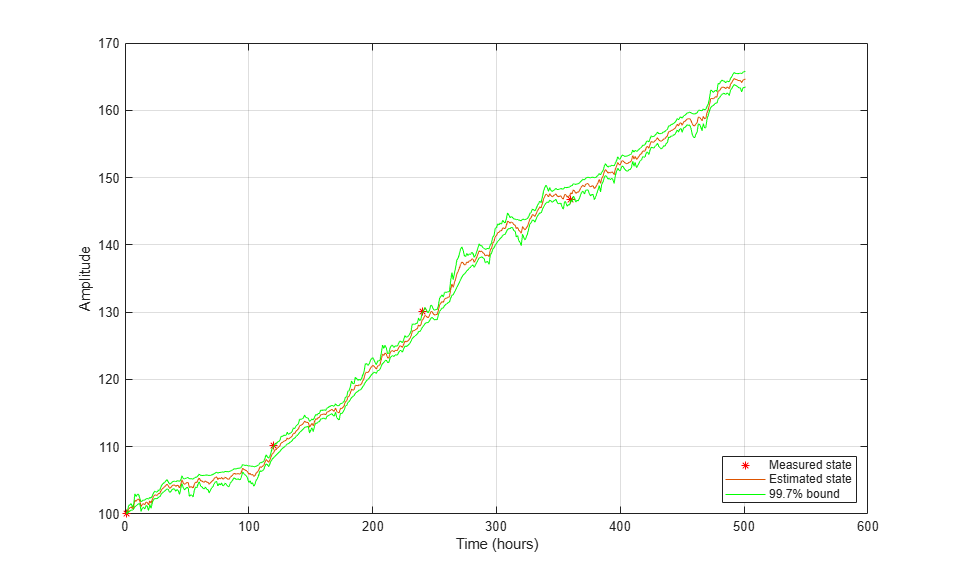Documentation

## Time Series Prediction and Forecasting for Prognosis

This example shows how to create a time series model and use the model for prediction, forecasting, and state estimation. The measured data is from an induction furnace whose slot size erodes over time. The slot size cannot be measured directly but the furnace current and consumed power are measured. It is known that as the slot size increases, the slot resistance decreases. The ratio of measured current squared to measured power is thus proportional to the slot size. You use the measured current-power ratio (both current and power measurements are noisy) to create a time series model and use the model to estimate the current slot size and forecast the future slot size. Through physical inspection the induction furnace slot size is known at some points in time.

### Load and Plot the Measured Data

The measured current-power ratio data is stored in the `iddata_TimeSeriesPrediction` MATLAB file. The data is measured at hourly intervals and shows that over time the ratio increases indicating erosion of the furnace slot. You develop a time series model using this data. Start by separating the data into an identification and a validation segment.

```load iddata_TimeSeriesPrediction n = numel(y); ns = floor(n/2); y_id = y(1:ns,:); y_v = y((ns+1:end),:); data_id = iddata(y_id, [], Ts, 'TimeUnit', 'hours'); data_v = iddata(y_v, [], Ts, 'TimeUnit', 'hours', 'Tstart', ns+1); plot(data_id,data_v) legend('Identification data','Validation data','location','SouthEast'); ```### Model Identification

The slot erosion can be modelled as a state-space system with noise input and measured current-power ratio as output. The measured current-power ratio is proportional to the system state, or

``
``

Wherethe state vector, contains the slot size;is the measured current-power ratio;noise andare to be identified.

Use the `ssest()` command to identify a discrete state-space model from the measured data.

```sys = ssest(data_id,1,'Ts',Ts,'form','canonical') ```
```sys = Discrete-time identified state-space model: x(t+Ts) = A x(t) + K e(t) y(t) = C x(t) + e(t) A = x1 x1 1.001 C = x1 y1 1 K = y1 x1 0.09465 Sample time: 1 hours Parameterization: CANONICAL form with indices: 1. Disturbance component: estimate Number of free coefficients: 2 Use "idssdata", "getpvec", "getcov" for parameters and their uncertainties. Status: Estimated using SSEST on time domain data "data_id". Fit to estimation data: 67.38% (prediction focus) FPE: 0.09575, MSE: 0.09348 ```

The identified model minimizes the 1-step ahead prediction. Validate the model using a 10 step ahead predictor, i.e., givenuse the model to predict. Note that the error between the measured and predicted values,, are used to make theprediction.

Use the 10 step ahead predictor for the identification data and the independent validation data.

```nstep = 10; compare(sys,data_id,nstep) % comparison of 10-step prediction to estimation data grid('on'); ``````figure; compare(sys,data_v,nstep) % comparison to validation data grid('on'); ```The above exercise Both data sets show that the predictor matches the measured data.

Forecasting is used to further verify the model. Forecasting uses the measured data recordto compute the model state at time step n. This value is used as initial condition for forecasting the model response for a future time span. We forecast the model response over the time span of the validation data and then compare the two. We can also compute the uncertainty in forecasts and plot +/- 3 sd of their values.

```MeasuredData = iddata(y, [], Ts, 'TimeUnit', 'hours'); % = [data_id;data_v] t0 = MeasuredData.SamplingInstants; Horizon = size(data_v,1); % forecasting horizon [yF, ~, ~, yFSD] = forecast(sys, data_id, Horizon); % Note: yF is IDDATA object while yFSD is a double vector t = yF.SamplingInstants; % extract time samples yFData = yF.OutputData; % extract response as double vector plot(MeasuredData) hold on plot(t, yFData, 'r.-', t, yFData+3*yFSD, 'r--', t, yFData-3*yFSD, 'r--') hold off title('Forecasted response over the validation data''s time span') grid on ```The plot shows that the model response with confidence intervals (indicated by the red colored dashed curves) overlap the measured value for the validation data. The combined prediction and forecasting results indicate that the model represents the measured current-power ratio.

The forecasting results also show that over large horizons the model variance is large and for practical purposes future forecasts should be limited to short horizons. For the induction furnace model a horizon of 200 hours is appropriate.

Finally we use the model to forecast the response 200 steps into future for the time span of 502-701 hours.

```Horizon = 200; % forecasting horizon [yFuture, ~, ~, yFutureSD] = forecast(sys, MeasuredData, Horizon); t = yFuture.SamplingInstants; % extract time samples yFutureData = yFuture.OutputData; % extract response as double vector plot(t0, y,... t, yFutureData, 'r.-', ... t, yFutureData+3*yFutureSD, 'r--', ... t, yFutureData-3*yFutureSD, 'r--') title('Forecasted response (200 steps)') grid on ```The blue curve shows the measured data that spans over 1-501 hours. The red curve is the forecasted response for 200 hours beyond the measured data's time range. The red dashed curves shows the 3 sd uncertainty in the forecasted response based on random sampling of the identified model.

### State Estimation

The identified model matches the measured current-power ratio but we are interested in the furnace slot size which is a state in the model. The identified model has an arbitrary state that can be transformed so that the state has meaning, in this case the slot size.

Create a predictor for the arbitrary state. The identified model covariances need to be translated to the predictor model using the `translatecov()` command. The `createPredictor()` function simply extracts the third output argument of the `predict()` function to be used with `translatecov()`.

```type createPredictor est = translatecov(@(s) createPredictor(s,data_id),sys) ```
```function pred = createPredictor(mdl,data) %CREATEPREDICTOR Return 1-step ahead predictor. % % sys = createPredictor(mdl,data) % % Create a 1-step ahead predictor model sys for the specified model mdl % and measured data. The function is used by % |TimeSeriedPredictionExample| and the |translatecov()| command to % translate the identified model covariance to the predictor. % Copyright 2015 The MathWorks, Inc. [~,~,pred] = predict(mdl,data,1); est = Discrete-time identified state-space model: x(t+Ts) = A x(t) + B u(t) y(t) = C x(t) + D u(t) A = x1 x1 0.9064 B = y1 x1 0.09465 C = x1 y1 1 D = y1 y1 0 Sample time: 1 hours Parameterization: CANONICAL form with indices: 1. Feedthrough: none Disturbance component: none Number of free coefficients: 2 Use "idssdata", "getpvec", "getcov" for parameters and their uncertainties. Status: Created by direct construction or transformation. Not estimated. ```

The model `est` is a 1-step ahead predictor expressed in the same state coordinates as the original model `sys`. How do we transform the state coordinates so that the model's state corresponds to the (time dependent) slot size? The solution is to rely on actual, direct measurements of the slot size taken intermittently. This is not uncommon in practice where the cost of taking direct measurements is high and only be done periodically (such as when the component is being replaced).

Specifically, transform the predictor state,, to, so thatwherethe measured current-power ratio, andis the furnace slot size. In this example, four direct measurements of the furnace slot size, `sizeMeasured`, and furnace current-power ratio, `ySizeMeasured`, are used to estimate. In transforming the predictor the predictor covariances also need to be transformed. Hence we use the `translatecov()` command to carry out the state coordinate transformation.

```Cnew = sizeMeasured\ySizeMeasured; est = translatecov(@(s) ss2ss(s,s.C/Cnew),est) ```
```est = Discrete-time identified state-space model: x(t+Ts) = A x(t) + B u(t) y(t) = C x(t) + D u(t) A = x1 x1 0.9064 B = y1 x1 0.9452 C = x1 y1 0.1001 D = y1 y1 0 Sample time: 1 hours Parameterization: CANONICAL form with indices: 1. Feedthrough: none Disturbance component: none Number of free coefficients: 2 Use "idssdata", "getpvec", "getcov" for parameters and their uncertainties. Status: Created by direct construction or transformation. Not estimated. ```

The predictor is now expressed in the desired state coordinates. It has one input that is the measured system output (the furnace current-power ratio) and one output that is the predicted system output (the furnace slot size). The predictor is simulated to estimate the system output and system state.

```opts = simOptions; opts.InitialCondition = sizeMeasured(1); U = iddata([],[data_id.Y; data_v.Y],Ts,'TimeUnit','hours'); [ye,ye_sd,xe] = sim(est,U,opts); ```

Compare the estimated output and slot size with measured and known values.

```yesdp = ye; yesdp.Y = ye.Y+3*ye_sd; yesdn = ye; yesdn.Y = ye.Y-3*ye_sd; n = numel(xe); figure, plot([data_id;data_v],ye,yesdp,'g',yesdn,'g') legend('Measured output','Estimated output','99.7% bound','location','SouthEast') grid('on') figure, plot(tSizeMeasured,sizeMeasured,'r*',1:n,xe,1:n,yesdp.Y/est.C,'g',1:n,yesdn.Y/est.C,'g'); legend('Measured state','Estimated state','99.7% bound','location','SouthEast') xlabel('Time (hours)') ylabel('Amplitude'); grid('on') ```### Using Prediction and Forecasting for Prognosis

The combination of predictor model and forecasting allow us to perform prognosis on the induction furnace.

The predictor model allows us to estimate the current furnace slot size based on measured data. If the estimated value is at or near critical values an inspection or maintenance can be scheduled. Forecasting allows us to, from the estimated current state, predict the future system behaviour allowing us to predict when an inspection or maintenance may be needed.

Further the predictor and forecast model can be re-identified as more data becomes available. In this example one data set was used to identify the predictor and forecast models but as more data is accumulated the models can be re-identified.